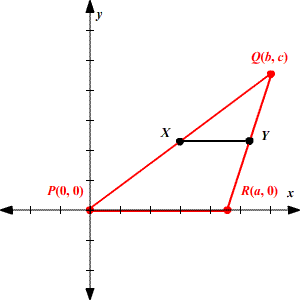# Coordinate Proofs

The coordinate proof is a proof of a geometric theorem which uses "generalized" points on the Cartesian Plane to make an argument.

The method usually involves assigning variables to the coordinates of one or more points, and then using these variables in the midpoint or distance formulas .

For example, the following is a coordinate proof of the Triangle Midsegment Theorem , which states that the segment connecting the midpoints of two sides of a triangle is parallel to the third side and exactly half the length.

Without loss of generality, we can assume that one side of the triangle lies on the $x$ -axis with one vertex at $\left(0,0\right)$ and the other vertex at $\left(a,0\right)$ .

Let the third vertex have the coordinates $\left(b,c\right)$ . We can assume without loss of generality that this third vertex lies in the first quadrant. (If it doesn't, you can just reflect the triangle over the $x$ - and/or $y$ -axis until it does.)To Prove: $\stackrel{¯}{XY}$ is parallel to $\stackrel{¯}{PR}$ and

$XY=\frac{1}{2}PR$

Proof:

Using the midpoint formula , the coordinates of the midpoints $X$ and $Y$ are

$\begin{array}{l}X\left(\frac{0+b}{2},\frac{0+c}{2}\right)=X\left(\frac{b}{2},\frac{c}{2}\right)\\ Y\left(\frac{a+b}{2},\frac{0+c}{2}\right)=Y\left(\frac{a+b}{2},\frac{c}{2}\right)\end{array}$

First note that the slope is $0$ , since

$\begin{array}{l}m=\frac{\text{rise}}{\text{run}}\\ \text{\hspace{0.17em}}\text{\hspace{0.17em}}\text{\hspace{0.17em}}\text{\hspace{0.17em}}\text{\hspace{0.17em}}=\frac{\left(\frac{c}{2}-\frac{c}{2}\right)}{\left(\frac{b}{2}-\left(\frac{a+b}{2}\right)\right)}\\ \text{\hspace{0.17em}}\text{\hspace{0.17em}}\text{\hspace{0.17em}}\text{\hspace{0.17em}}\text{\hspace{0.17em}}=\frac{0}{\left(\frac{b}{2}-\left(\frac{a+b}{2}\right)\right)}\\ \text{\hspace{0.17em}}\text{\hspace{0.17em}}\text{\hspace{0.17em}}\text{\hspace{0.17em}}\text{\hspace{0.17em}}=0\end{array}$

So, $\stackrel{¯}{XY}$ is parallel to $\stackrel{¯}{PR}$ .

Now, compare the lengths of the two segments.

$\begin{array}{l}XY=\frac{a+b}{2}-\frac{b}{2}\\ \text{\hspace{0.17em}}\text{\hspace{0.17em}}\text{\hspace{0.17em}}\text{\hspace{0.17em}}\text{\hspace{0.17em}}\text{\hspace{0.17em}}\text{\hspace{0.17em}}\text{\hspace{0.17em}}=\frac{a}{2}\\ PR=a-0\\ \text{\hspace{0.17em}}\text{\hspace{0.17em}}\text{\hspace{0.17em}}\text{\hspace{0.17em}}\text{\hspace{0.17em}}\text{\hspace{0.17em}}\text{\hspace{0.17em}}\text{\hspace{0.17em}}=a\end{array}$

So,

$XY=\frac{1}{2}PR$

This concludes the proof.